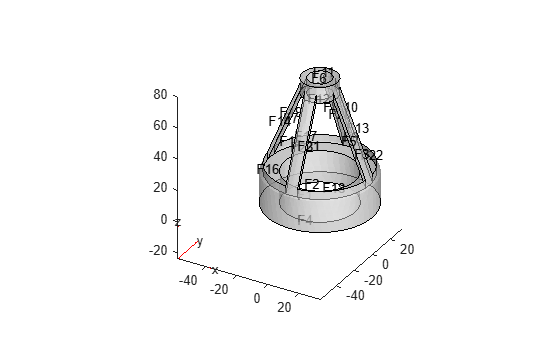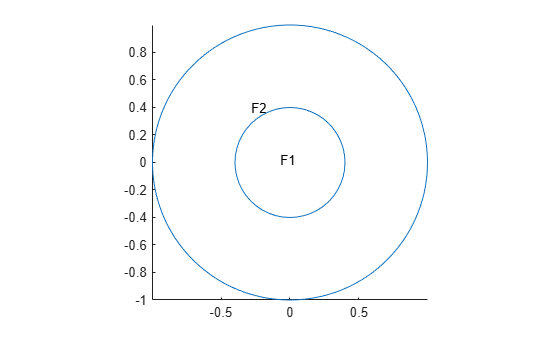## Geometry from Triangulated Mesh

### 3-D Geometry from a Finite Element Mesh

This example shows how to import a 3-D mesh into a PDE model. Importing a mesh creates the corresponding geometry in the model.

The `tetmesh` file that ships with your software contains a 3-D mesh. Load the data into your Workspace.

`load tetmesh`

Examine the node and element sizes.

`size(tet)`
```ans = 1×2 4969 4 ```
`size(X)`
```ans = 1×2 1456 3 ```

The data is transposed from the required form as described in `geometryFromMesh`.

Create data matrices of the appropriate sizes.

```nodes = X'; elements = tet';```

Create a PDE model and import the mesh.

```model = createpde(); geometryFromMesh(model,nodes,elements);```

The model contains the imported mesh.

`model.Mesh`
```ans = FEMesh with properties: Nodes: [3x1456 double] Elements: [4x4969 double] MaxElementSize: 8.2971 MinElementSize: 1.9044 MeshGradation: [] GeometricOrder: 'linear' ```

View the geometry and face numbers.

`pdegplot(model,'FaceLabels','on','FaceAlpha',0.5)`### 2-D Multidomain Geometry

Create a 2-D multidomain geometry from a mesh.

Load information about nodes, elements, and element-to-domain correspondence into your workspace. The file `MultidomainMesh2D` ships with your software.

`load MultidomainMesh2D`

Create a PDE model.

`model = createpde;`

Import the mesh into the model.

`geometryFromMesh(model,nodes,elements,ElementIdToRegionId);`

View the geometry and face numbers.

`pdegplot(model,'FaceLabels','on')`##### SupportGet trial now